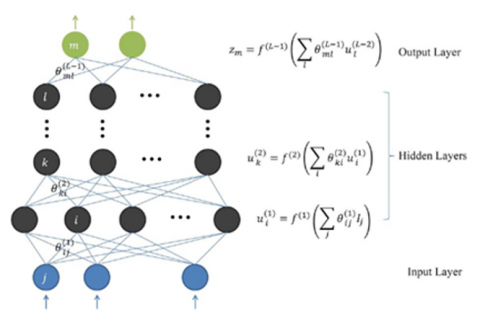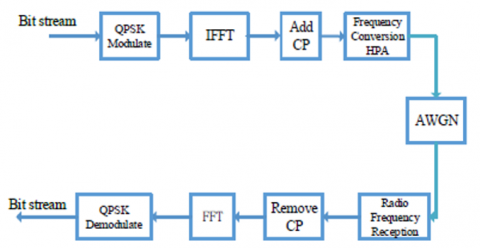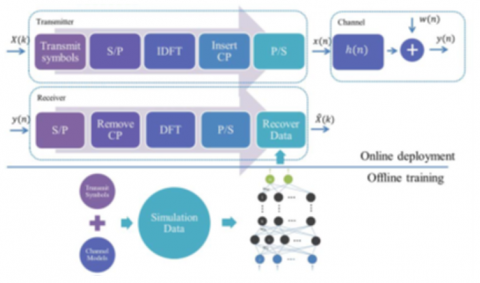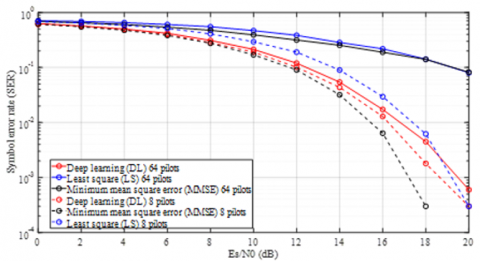# Effect of DNN Approximation for Channel Estimation and Signal Detection on OFDM Applications

Effect of DNN Approximation for Channel Estimation and Signal Detection on OFDM Applications
Electrical and Electronical Engineering, Firat University, Elazığ 23000, Turkey

Corresponding Author Email:
bkamislioglu@firat.edu.tr
Page:
2181-2185
|
DOI:
https://doi.org/10.18280/ts.390632
15 June 2022
|
Accepted:
10 October 2022
|
Published:
31 December 2022
| Citation

Special Issue: The 3rd ICAENS Conference

OPEN ACCESS

Special Issue
Abstract:

This paper offers a deep learning approximation to realize channel estimation and signal detection that creates the main communication structure skeleton for the orthogonal frequency-division multiplexing (OFDM) system known as an efficient modulation type on 5G. This letter offers an application of deep learning to handle the wireless OFDM channels' end-to-end conduct. First, channel state information (CSI) is predicted explicitly that differs from existing OFDM receivers, then detected the transmitted symbols utilizing the predicted CSI. In the end, CSI is predicted by the suggested deep learning approximation indirectly and transmitted symbols are directly recovered. The structure of the designed receiver occurs of a layer of DNN and soft decisions, which resolves the issues channel estimation error, time delay, and limitation of decoding between users in classic detection techniques. In the simulation results, it is observed that the receiver has powerful stability on the power distribution of user, not only convenient for the linear channel, but also for nonlinear channel when enhancement the number of users, also detection can be well on the receiver. Generally, the efficiency of the modulation system decreases with the features of the multipath channel utilized for transmission. Channel estimation and detection of symbols utilize to reduce the impacts of the channel, which needs high computation and bandwidth conventionally. This paper is used deep neural networks (DNN) for detecting the signal, in this way much effort in detecting the channel is prevented. The proposed method saves priceless bandwidth via used CP in OFDM with a big increase in SNR.

Keywords:

OFDM, DNN, signal detection, modulation system

1. Introduction

Orthogonal Frequency Division Multiplexing has been a great focus of interest in designing to generate waveforms for the next-generation 5G wireless network . The main components of 5G radio which are New Radio (NR) and Long Term Evolution (LTE) prefer Cyclic Prefix OFDM (CP-OFDM) to do modulation best fitted. 5G prefers the OFDM modulation technique as it obtains low complexity on transmitter and receiver, higher spectral efficiency in MIMO, lower power expense, and better capacity in frequency localization.

In communication systems, frequency selective fading of channels may outcome in very desperate BERs. OFDM utilizes orthogonal subcarriers transporting data modulated with any digital modulation methods available. Due to separate subcarriers, the frequency selective fading converts to flat fading so there is a reduction of desperate impacts. As each subcarrier has exact multiples of the principal constituent this situation makes them orthogonal to one other. Channel delay dispersions cause Inter Symbol Interference (ISI) and non-orthogonal received signals cause Inter-Carrier Interference (ICI) and these are relieved by the supplement of a CP. Guard intervals are revealed adding unused subcarriers.

As signals are transmitted, usually deteriorated by the effects of the channel and these effects reduces by using channel equalization methods.

As a popular modulation scheme, orthogonal frequency-division multiplexing (OFDM) has embraced widely to overcome fading of frequency selectivity in wireless channel communications. In OFDM system, known as channel state information (CSI) is an important value for consistent detection and decoding. The pilots can estimate CSI before the detection of the transmitted data normally, and transmitted symbols can rescue at the receiver using the estimated CSI [2-6].

The traditional estimation of channel techniques, Minimum Mean-Square Error (MMSE) and Least Squares (LS) have been used and made optimized for lots of different situations . The LS estimation method utilizes no previous statistics of the channel, so its yield may be deficient. The MMSE estimation method generally obtains greatly better success of detection via using the channels statistics of second-order.

This work introduces a deep learning approximation to the estimation of channels and detection of symbol for an OFDM system. There is numerous applications area on artificial neural networks (ANNs) and deep learning, especially, channel equalization , and channel decoding  has applies based on CSI  successfully in communication applications. ANNs set the coefficients with respect to the pilot data, but since the number of parameters increases a lot in deep neural networks (DNNs), these methods cannot be implemented straightly and conclude a great number to train data and an extended training time [11-14]. Without calculation of the channel matrix, the store and universalization features of deep learning are verified productive in signal estimation .

In this work, it is explicit that the suggested method clarifies the impact of multipath upon Bit Error Rate (BER), also obvious that helps to obtain high-truth digital systems. Since wireless communication resources are limited, efficient use of bandwidth is the main goal of communication technology, thus in this signal detection method, even with no cyclic prefix, the BER is not much impressed which assists to save valued bandwidth. Obtained results from the end of this work, the deep learning approximation confirms has more sturdy features than LS and MMSE where trained pilot symbols are reduced, and the CP has been removed.

The main lines of this paper plan as follows. The deep learning structure and OFDM structure are given in Section II. Applications includes conclusion of the simulation for detection of signal in the OFDM structure is presented in Section III. Section IV finalizes the paper.

2. Materials and Method

Deep learning and OFDM structure are determined in this section.

2.1 Deep learning structure

DNNs need a huge count of data and need to train before they are implemented offline. DNNs utilize a huge count of data and need to train before they are implemented offline. Therefore, there are two phases of distributing the DNN; the first phase supposes training as offline and the other phase supposes online distribution of the training net . As we need a big number of data on the training, a channel that known model is anticipated to be utilized. Models of developed channels can define the realistic channels efficiently when full statistics of channels are given. To simulate and generate a big number of data with changing statistics of the channel is made easy by using the developed channel models.

## 111.pngFigure 1. Deep learning structure

The pilot in OFDM symbols and the data in OFDM symbol came to DNN as inputs. The pilot and data symbols together are discretized into imaginary parts and real parts and applied network as input and so the count of input neurons can reproduce to be 256 neurons when we use 64 subcarriers. The output layer of DNN estimates and recovers the received data as bits and the Sigmoid activation function is used in this layer. As given Table 1 DNN parameters are batch size, number of class, max epochs, number of hidden units and input size.

Table 1. Training parameters of DNN

 Parameter batch size number of class epoch number of hidden input size Value 1000 4 100 16 256

Deep learning applications have been successful in a large variety of fields via an important success developed, including opinion on computers, the processing of inherent language, speech identification and so on. Generally, DNNs that present deepness sample of ANNs raise the hidden layers' number to develop the ability to identify. In the network each layer is formed of multiple neurons, which is a nonlinear function of a summation of the neurons on the previous phase and illustrated in Figure 1.

Sigmoid and Relu functions can specify as the nonlinear function, determined as fk(x)=1/(1+e−x), and fl(x)=max(0,x). So, through a series of non-linear transforms of input data (I) is formed the network output w is stated as Eq. (1) mathematically .

$z=f(D, Q)=f^{(D-1)} f^{(D-2)}\left(\ldots f^{(1)}(D)\right)$                        (1)

In Eq. (1), layers the number is D, and the neural networks’ weights are stood by Q. The weights on the neurons are defined as the parameters of the model, and on a training set, the optimal weights are usually obtained using acknowledged wanted outputs .

2.2 OFDM structure

In the transmitter block, the bit flow is corresponded to a matrix of dimension Nt × Nf, if the count of antennas on the transmitter side is assumed Nt and the count of subcarriers is assumed Nf. Figure 2 shows a block of an OFDM system under nonlinear distortion. In the process of data transmission first, any modulation is performed, then Inverse Fast Fourier Transform (IFFT) is implemented, and then a CP is added, and finally, the RF is amplified and sent the data. The transmitted signal has non-linearity distorted owing to the amplifier. The matrix includes the QAM (Quadrature Amplitude Modulation) or PSK (Phase-Shift Keying) symbols, all row indicates an antenna mark, and all column shows a subcarrier. Then each row modulates with OFDM utilizing traditional techniques .

## 2.pngFigure 2. Block diagram of OFDM

In Figure 2, the OFDM structure, the transmission channel is regarded to keep non-changed for two successive OFDM symbols, one of these includes generally pilots, while the others contain the data, and these two symbols are jointly described as a frame. The pilot location should maintain stability along all steps of the training and propagation to realize estimation of channel effectively. When the signal has been carried throughly the channel and accepted by the receiver antenna, the transmitted symbol is transformed into a parallel data flow thus the CP is extracted and FFT operation is applied .

The receiver side makes the inverse processes of the transmit side. First, receiver removes the cyclic prefix and then transformation of FFT and demodulation processes are implemented, and finally, an original bit stream is obtained.

Considering multi path channel defined with complicated random factors {h(m)}(m=0….,M−1),  the received signal y(m) clarifies in Eq. (2).

$y(m)=x(m) \otimes h(m)+w(m)$                        (2)

Eq. (2) shows the convolution as circular between x(m) is described transmitted signal and h(m) is defined channel response and w(m) is introduced the AWGN (Additive White Gaussian Noise) is added to this.

$Y(k)=X(k) H(k)+W(k)$                        (3)

The receiving signal on the frequency area is given with Eq. (3) following extracting the CP and enforcing the DFT process and the DFT of y(m), x(m), h(m) and w(m) are Y, X, H, and W. The receiving data symbols formed a pilot build, a data build is assumed as input in our work, and the transmitted data is recovered by the purposed DNN method.

Equation 3 can write as vector form in Eq. (4).

$\bar{Y}=X \bar{H}+\bar{W}$                        (4)

When X is assumed a matrix of input, $\bar{Y}$  is assumed output matrix, cost function in LS Channel Estimation can be written as Eq. (5).

$C=\|\bar{Y}-X \bar{H}\|^2$                        (5)

$\hat{H}_{L S}=\left(X^H X\right)^{-1} X^H Y$                        (6)

The solution of LS estimators is given in Equation 6 and because of invertible X, Equation 6 can rewrite as Eq. (7) as a conclusion Eq. (8) is obtained.

$\hat{H}_{L S}=\left(X^H\right)^{-1} X^{-1} X^H Y$                       (7)

$\hat{H}_{L S}=X^{-1} Y$                      (8)

LS estimators have the advantage of their simplicity and make calculations with very low complexity with no information about statistics of the channel.

The mean value of coefficients of channel can define as E{H(k)}=0 and variance can determine as $E\left\{|H(k)|^2\right\}=L \sigma_h^2$ . Also, the mean and variance of AWGN channel can write as E{W(k)}=0 and $E\left\{|W(k)|^2\right\}=N \sigma_n^2$  respectively.

L assumes number of multi-paths and N assumes number of subcarriers. Channel and noise variances are given by $\sigma_h$  and $\sigma_n$  respectively. MMSE estimators are defined in Eq. (9).

$\hat{H}_{M M S E}$ $(k)=R_{H(k) Y(k)}$ $R_{Y(k) Y(k)}$ $Y(k)$                       (9)

Cross covariance of H(k) and Y(k) are illustrated with $R_{H(k) Y(k)}$  and given in Eqns. (10) and (11).

$R_{H(k) Y(k)}=E\left\{H(k) Y^*(k)\right\}=L \sigma_h^2 X^*(k)$                      (10)

$R_{Y(k) Y(k)}=E\left\{Y(k) Y^*(k)\right\}$$=L \sigma_h^2\left|X^*(k)\right|^2 N \sigma_n^2 (11) \hat{H}_{M M S E} (k)$$=L \sigma_h^2 X^*(k) \frac{1}{L \sigma_h^2|X(k)|^2+N \sigma_n^2} Y(k)$                      (12)

MMSE channel estimation equation is given in Eq. (12) by using Eqns. (10) and (11).

There are two stages as shown in Figure 3 to do an efficient DNN application on both symbol detection and channel estimation. For the offline training phase, we train the model with the received OFDM symbols, which are created with diverse data sequences, and for different channel cases. For the online spreading phase, the DNN approximation creates the output, which rescues the transmitting data with no estimate of the wireless channel clearly.

## 3.pngFigure 3. Online deployment and Offline training of OFDM block diagram

Many channel models have improved that define the real channels greatly considering statistics of channel. The training data is acquired using these channel models by simulation. In all steps of the simulation, first, we generate a random data sequence for the transmitted signals and compose the matching OFDM skeleton with a pilot symbol series. Then we simulate the available random channel related to the models of the channel. OFDM signal that is received includes the valid distortion of the channel and the noise of channel based on the OFDM skeleton. The training data is collected from the receiver symbol and the actually transmitter symbol. The receiver symbol from the pilot build and one data build is admitted as the input of the pattern of deep learning. To make smaller the gap among the neural network output and the transmitting symbol, the model of deep learning is trained [17-21].

3. Applications

Several experiments were implemented to indicate the deep learning methods' performance in both estimation of the channel and detection of the symbol in OFDM communication systems. Based on simulated data a DNN model has been trained and a comparison has been realized with the conventional methods, related to BER (Bit-Error Rate) and different SNR (Signal-to-Noise Ratio).

Applications are realized using 64 subcarriers and the CP of lengths 16 and 8 in the purposed OFDM system and QPSK is selected as the modulation technique. This paper presents a comparison between the proposed method via the LS and MMSE estimation techniques for estimating of the channel and detecting of the channel, when 64 and 8 pilots are utilized to make estimation to channel in each cycle.

## 4.pngFigure 4. Accuracy of trained model and loss of trained model

Firstly, trained of DNN process is operated and accuracy and loss of the trained model diagrams are illustrated in Figure 4. In Figure 5, when compared to the performance of methods, the LS estimator shows a very poor output since there is no past statistics knowledge of the channel to detect.

## 5.pngFigure 5. SER to SNR for DL, LS and MMSE including 64 and 8 pilots

Conversely, the MMSE approximation indicates the top success because the statistics of the second order of the channels utilized for symbol detection are accepted to known. It is seen that the proposed deep learning approximation shows a considerable preferable success than the LS technique and can compare to the MMSE technique. In Figure 5 for used 8 pilots, the obtained BER curves of using the LS and MMSE techniques arrive at a satiate when SNR exceeds 10 dB while in the deep learning technique BER reduces with increasing SNR. This situation shows that the DNN has sturdy features to the count of pilots utilized in the estimation of the channel. DNN has excellent performance because the features of the radio channels knows based upon the trained data formed from the pattern. We need CP to make turning the convolution method linear to circular of the somatic channel and reduce ISI. However, using CP leads to expenses extra time and energy to transmit data. The BER curves are illustrated in Figure 6 for an OFDM system without CP and it is clear that neither MMSE nor LS techniques can efficiently predict the channel.

## 6.pngFigure 6. SER curves for with CP and without CP

However, the deep learning technique operates quite well which shows the DNNs bring to light and learn features of the wireless channel in the training cycle.

4. Conclusions

In this work, a DNN structure is trained utilizing simulated data, and a comparison is carried out with the conventional techniques in regard to BER for different SNR. Also impact of pilot symbol number, impact of CP and impact of robustness are examined.  In the BER graphs that used 8 pilots on LS and MMSE techniques a saturation is traced when SNR value has more than 10 dB, the deep learning based technique still demonstrates the capability to decrease the BER via improving SNR this shows that the DNN demonstrates robustness to number of pilot symbol utilized for an estimate of the channel. DNN has excellent performance because the features of the radio channels knows based upon the trained data formed from the pattern. In OFDM system without CP, it is clear that neither MMSE nor LS techniques can efficiently predict the channel however, the deep learning technique operates quite well.

In this paper, the deep learning approximation is verified to be sturdy features than LS and MMSE, which trained pilot symbols are reduced, the CP has been removed. This paper has presented DNNs for symbol detection and channel estimation in an OFDM system. As seen in the results of simulation, the deep learning technique has great advance considering radio channels have made difficult interference and distortion. It is also concluded that DNNs can recall and examine the confused features of wireless channels. Having a good generalization capability of the DNN model is important for real-world applications because while the circumstances of online spread are not precisely compliant with the used models of channel in the training step, DNN can still operate efficiently. Results of simulation show that the purposed DNN has superior capable to decrease in the overhead of pilot symbols and adding CP, in spite of the traditional techniques could not.  In future work, samples of the real radio channels can be gathered to train again or sensitive the model to obtain superior success.

References

 Rezgui, C., Grayaa, K. (2016). An enhanced channel estimation technique with adaptive pilot spacing for OFDM system. In 2016 International Symposium on Networks, Computers and Communications (ISNCC), pp. 1-4. https://doi.org/10.1109/ISNCC.2016.7746098

 Abdelgader, A.M.S., Feng, S., Wu, L. (2017). On channel estimation in vehicular networks. IET Communications, 11(1): 142-149. https://doi.org/10.1049/iet-com.2016.0577

 Ye, H., Li, G.Y., Juang, B.H. (2017). Power of deep learning for channel estimation and signal detection in OFDM systems. IEEE Wireless Communications Letters, 7(1): 114-117. https://doi.org/10.1109/LWC.2017.2757490

 Wang, X., Gao, L., Mao, S., Pandey, S. (2016). CSI-based fingerprinting for indoor localization: A deep learning approach. IEEE Transactions on Vehicular Technology, 66(1): 763-776. https://doi.org/10.1109/TVT.2016.2545523

 Nachmani, E., Be'ery, Y., Burshtein, D. (2016). Learning to decode linear codes using deep learning. In 2016 54th Annual Allerton Conference on Communication, Control, and Computing (Allerton), pp. 341-346. https://doi.org/10.1109/ALLERTON.2016.7852251

 Chen, S., Gibson, G.J., Cowan, C.F.N., Grant, P.M. (1990). Adaptive equalization of finite non-linear channels using multilayer perceptrons. Signal Processing, 20(2): 107-119. https://doi.org/10.1016/0165-1684(90)90122-F

 Li, Y.L.J.C., Cimini, L.J., Sollenberger, N.R. (1998). Robust channel estimation for OFDM systems with rapid dispersive fading channels. IEEE Transactions on Communications, 46(7): 902-915. https://doi.org/10.1109/26.701317

 Cavers, J.K. (1991). An analysis of pilot symbol assisted modulation for Rayleigh fading channels (mobile radio). IEEE Transactions on Vehicular Technology, 40(4): 686-693. https://doi.org/10.1109/25.108378

 Huang, Q., Zhao, C., Jiang, M., Li, X., Liang, J. (2018). Cascade-Net: A new deep learning architecture for OFDM detection. arXiv preprint arXiv:1812.00023.

 Zaidi, A.A., Baldemair, R., Molés-Cases, V., He, N., Werner, K., Cedergren, A. (2018). OFDM numerology design for 5G new radio to support IoT, eMBB, and MBSFN. IEEE Communications Standards Magazine, 2(2): 78-83. https://doi.org/10.1109/MCOMSTD.2018.1700021

 Schmidhuber, J. (2015). Deep learning in neural networks: An overview. Neural Networks, 61: 85-117. https://doi.org/10.1016/j.neunet.2014.09.003

 Krizhevsky, A., Sutskever, I., Hinton, G.E. (2012). Imagenet classification with deep convolutional neural networks. Advances in Neural Information Processing Systems, 1097-1105.

 Kyosti, P. (2007). WINNER II channel models. Eur. Commission, Brussels, Belgium, Tech. Rep. D1.1.2 IST-4-027756-WINNER, Sep.

 Bhange, D., Dethe, C. (2020). performance optimization of ls/lmmse using swarm intelligence in 3d mimo-ofdm systems. Traitement du Signal, 37(1): 107-112. https://doi.org/10.18280/ts.370114

 Badi, A.M., Elganimi, T.Y., Alkishriwo, O.A., Adem, N. (2021). Channel estimation based on machine learning paradigm for spatial modulation OFDM. In 2021 IEEE 1st International Maghreb Meeting of the Conference on Sciences and Techniques of Automatic Control and Computer Engineering MI-STA, pp. 752-755. https://doi.org/10.1109/MI-STA52233.2021.9464456

 Zhang, J., Wen, C.K., Jin, S., Li, G.Y. (2019). Artificial intelligence-aided receiver for a CP-free OFDM system: Design, simulation, and experimental test. IEEE Access, 7: 58901-58914. https://doi.org/10.1109/ACCESS.2019.2914928

 Yechuri, P.K., Ramadass, S. (2021). Classification of image and text data using deep learning-based LSTM model. Traitement du Signal, 38(6): 1809-1817. https://doi.org/10.18280/ts.380625

 Shi, W., Zhao, C., Jiang, M. (2019). Reduced complexity interference cancellation for OFDM systems with insufficient cyclic prefix. In 2019 11th International Conference on Wireless Communications and Signal Processing (WCSP), pp. 1-6. https://doi.org/10.1109/WCSP.2019.8928022

 Marseet, A.A., Elganimi, T.Y. (2019). Fast detection based on customized complex valued convolutional neural network for generalized spatial modulation systems. In 2019 IEEE Western New York Image and Signal Processing Workshop (WNYISPW), pp. 1-5. https://doi.org/10.1109/WNYIPW.2019.8923057

 Xiang, L., Liu, Y., Van Luong, T., Maunder, R.G., Yang, L.L., Hanzo, L. (2020). Deep-learning-aided joint channel estimation and data detection for spatial modulation. IEEE Access, 8: 191910-191919. https://doi.org/10.1109/ACCESS.2020.3032627

 Sutar, M.B., Patil, V.S. (2017). LS and MMSE estimation with different fading channels for OFDM system. In 2017 International conference of Electronics, Communication and Aerospace Technology (ICECA), 1: 740-745. https://doi.org/10.1109/ICECA.2017.8203641